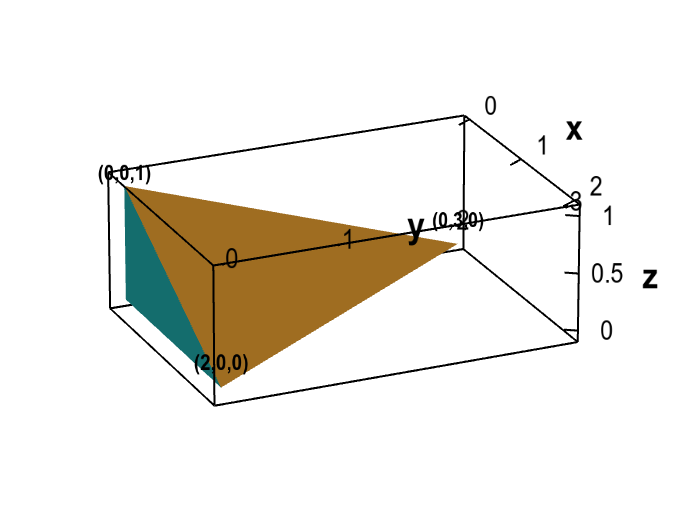# Math Insight

### Applet: A tetrahedronThe tetrahedron is bounded by the coordinate planes ($x=0$, $y=0$, and $z=0$) and the plane through the three points (2,0,0), (0,3,0), and (0,0,1).